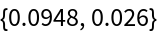Function Repository Resource:

Pairs each element of a list with its index

Contributed by: Sander Huisman
 ResourceFunction["AddIndices"][{e1,e2,…}] pairs each ei with its index i forming the list {{1,e1},{2,e2},…}. ResourceFunction["AddIndices"][{e1,e2,…},imin] pairs each ei with its index starting from imin forming the list {{imin,e1},{imin+1,e2},…}. ResourceFunction["AddIndices"][{e1,e2,…},imin,di] uses step di forming the list {{imin,e1},{imin+di,e2},…}.

Details and Options

Frequently used for when a large list of items needs to be transformed but the original indices have to be preserved.

Examples

Basic Examples

Add indices to a list of elements:

 In:=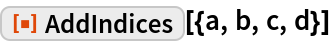Out=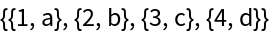Scope

 In:=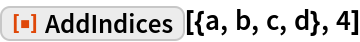Out=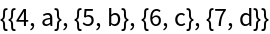The starting index and the step can be symbolic. Start the index at x:

 In:=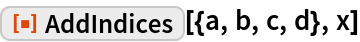Out=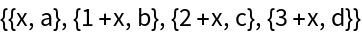The step can also be symbolic:

 In:=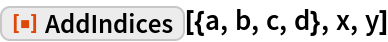Out=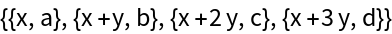Applications

Select palindromic words from a Select while retaining their indices:

 In:=Out=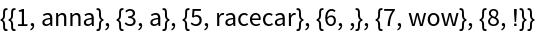Sort the strings and retain their original indices:

 In:=Out=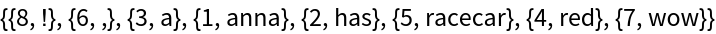Properties and Relations

AddIndices can be achieved using MapIndexed:

 In:=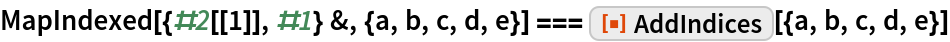Out=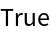AddIndices is roughly 4 times faster:

 In:=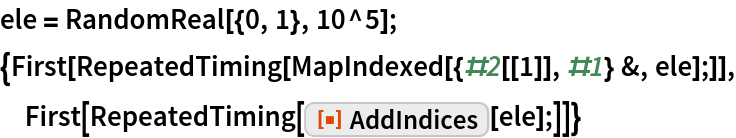Out=